# How to Generate Random Time in Excel

In some special situations we may need to generate some random times in worksheet. We can enter time manually and one by one, but if we want to require a lot of random dates in excel worksheet, we will spend a lot of time on manual entry, and this work is very boring. Above all, we need to find out a quick and convenient way to insert random times in excel, this tutorial will help you to solve this problem by enter formula with TEXT and RAND functions.

## Part 1: Generate Random Time in Excel

Step 1: Select one cell, enter the formula =TEXT(RAND(),”HH:MM:SS”). RAND function returns a random number, TEXT function can convert number to a proper format, in this formula we convert number to HH:MM:SS format.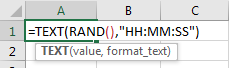Step 2: Click Enter to get the result. Verify that a random time is displayed.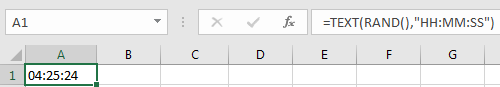Step 3: We just need to drag fill handle down to create more random times. You can also copy this formula to other cells to get more random times.

Comment:

Actually, you can also only use RAND function itself to get a random time here. For example, enter =RAND() in cell A1. Then we get a real number.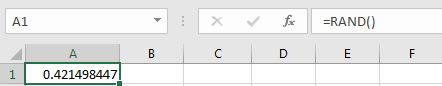In Home ribbon, click General dropdown list in Number group.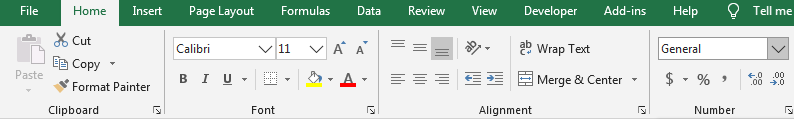Select Time format.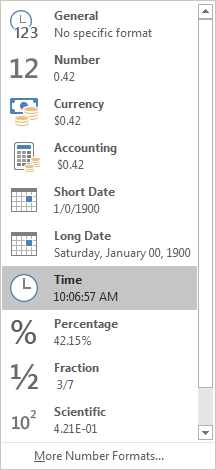Then we will get a random time properly.

## Part 2: Generate Random Time Between Two Times in Excel

Step 1: For RAND function, if you want to generate a number between a and b, we can use =RAND()*(b-a)+a to get a random number. So if we want to get a random time between a and b, we can use formula =TEXT(RAND()*(b-a)/24+a/24,”HH:MM:SS”). So, if we want get a random time between 7 to 13 o’clock, in cell A1, enter =TEXT(RAND()*(13-7)/24+7/24,”HH:MM:SS”).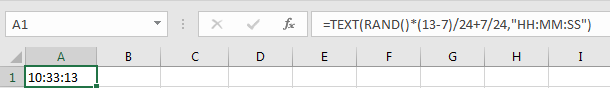In above screenshot, we can see that returned random time 10:33:13 can meet our expectation. As we mentioned above, we can also only use RAND function here as well, enter =RAND()*(13-7)/24+7/24, then convert it to time format.

Step 2: Drag fill handle down. Verify that random times between time 7 and 13 o’clock are displayed properly.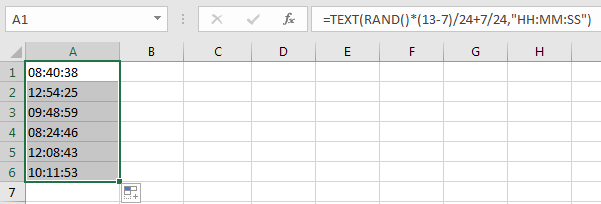## Part 3: Generate Random Date and Time in Excel

Step 1: Refer to above parts, we can update the formula to get date and time properly. Enter the formula

=TEXT(RAND()*(“1/1/2019 7:00:00” – “12/31/2019 13:00:00″)+”12/31/2019 13:00:00″,”MM/DD/YYYY HH:MM:SS”).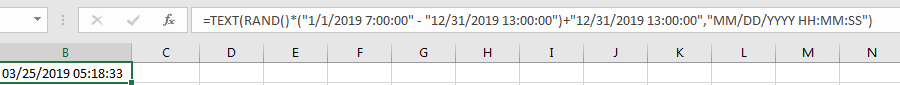Verify that a random date and time is returned properly. You can change start and end date time to meet your demands.

### Related Functions

• Excel Text function
The Excel TEXT function converts a numeric value into text string with a specified format. The TEXT function is a build-in function in Microsoft Excel and it is categorized as a Text Function. The syntax of the TEXT function is as below: = TEXT (value, Format code)…
• Excel RAND function
The Excel RAND function returns a random real number that is greater than or equal to 0 and less than 1. The syntax of the RAND function is as below:=RAND ()…

Related Posts

To pad a number with zeros, you need to add leading spaces and/or carriers. For example, if the original digit contains 2 digits, three additional characters will be added on before it. If there are 3 digits in total, allocating ...

How to Auto Fill Weekdays or Weekends in Excel

Sometimes we may want to enter a sequence of days in excel for some purpose, and we can press Ctrl+; to insert current date into cell, and then drag the cell down to attach following days into other cells, then ...

How to Change Date to The Day of Week in Excel

In excel, we can type a date properly if set cell format as ‘Date’. If there is a list includes multiple dates, is there any way to convert these dates to the days of week accordingly? Actually, there are some ...

How to Create Increment Number with Texts in Excel

Sometimes we want to fill 1,2,3,4,5… into each cell in a column, for implement this we can enter 1 in the first cell and then drag fill handle down to fill the following cells. But if there are some texts ...

How to Combine Text and Date into one Cell in Excel

This post will guide you how to combine text and dates in the same cell in Excel. How do I combine text and date values into one Cell in Excel 2013/2016. Combine Text and Date into One Cell Assuming that ...

How to Highlight Cell or Row If Date Is In Current Day/Week/Month in Excel

This post will guide you how to highlight cell if date is the current day or is in the current week or month in Excel. How do I highlight row if date is in current week or month with conditional ...

How to Convert Military Time to Standard Time in Excel

This post will guide you how to convert military time to standard time with a formula in Excel. How do I convert from military time 17:29:30 to regular time 5:29:30PM in Excel.  How do I Convert Standard time to military ...

How to Delete or Remove Year from a Date in Excel

This post will guide you how to delete or remove year from a given standard date in Excel. How do I remove the year from a date with a formula in Excel. Remove Year from a Date Assuming that you ...

How to Convert Date to YYYY-MM-DD format in Excel

This post will guide you how to convert the current date to a specified date format in Excel. How do I convert date to YYYY-MM-DD format with Format Cells Feature in Excel. How to convert date format to a specific ...

How to Concatenate Cells and keeping Date Format in Excel

This post will guide you how to concatenate cells and keeping data format in Excel. How do I concatenate cells and retain the certain Date format with a formula in Excel 2010/2013/2016. Concatenate Cells and Keeping Date Format Assuming that ...

Sidebar